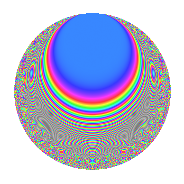# Properties

 Label 430.2.jLevel 430 Weight 2 Character orbit j Rep. character $$\chi_{430}(49,\cdot)$$ Character field $$\Q(\zeta_{6})$$ Dimension 44 Newform subspaces 1 Sturm bound 132 Trace bound 0

# Related objects

## Defining parameters

 Level: $$N$$ = $$430 = 2 \cdot 5 \cdot 43$$ Weight: $$k$$ = $$2$$ Character orbit: $$[\chi]$$ = 430.j (of order $$6$$ and degree $$2$$) Character conductor: $$\operatorname{cond}(\chi)$$ = $$215$$ Character field: $$\Q(\zeta_{6})$$ Newform subspaces: $$1$$ Sturm bound: $$132$$ Trace bound: $$0$$

## Dimensions

The following table gives the dimensions of various subspaces of $$M_{2}(430, [\chi])$$.

Total New Old
Modular forms 140 44 96
Cusp forms 124 44 80
Eisenstein series 16 0 16

## Trace form

 $$44q - 44q^{4} - 4q^{5} + 2q^{6} + 20q^{9} + O(q^{10})$$ $$44q - 44q^{4} - 4q^{5} + 2q^{6} + 20q^{9} + 8q^{11} + 4q^{14} - 4q^{15} + 44q^{16} - 4q^{19} + 4q^{20} - 24q^{21} - 2q^{24} + 12q^{26} - 10q^{29} - 20q^{31} - 12q^{34} + 12q^{35} - 20q^{36} + 120q^{39} + 20q^{41} - 8q^{44} - 28q^{45} + 42q^{49} - 112q^{51} - 68q^{54} - 26q^{55} - 4q^{56} + 40q^{59} + 4q^{60} + 8q^{61} - 44q^{64} - 60q^{65} - 12q^{66} - 4q^{69} + 48q^{70} - 20q^{71} - 12q^{74} + 4q^{75} + 4q^{76} - 44q^{79} - 4q^{80} + 2q^{81} + 24q^{84} + 20q^{85} + 14q^{86} - 26q^{89} + 68q^{90} + 4q^{94} - 34q^{95} + 2q^{96} + 72q^{99} + O(q^{100})$$

## Decomposition of $$S_{2}^{\mathrm{new}}(430, [\chi])$$ into newform subspaces

Label Dim. $$A$$ Field CM Traces $q$-expansion
$$a_2$$ $$a_3$$ $$a_5$$ $$a_7$$
430.2.j.a $$44$$ $$3.434$$ None $$0$$ $$0$$ $$-4$$ $$0$$

## Decomposition of $$S_{2}^{\mathrm{old}}(430, [\chi])$$ into lower level spaces

$$S_{2}^{\mathrm{old}}(430, [\chi]) \cong$$ $$S_{2}^{\mathrm{new}}(215, [\chi])$$$$^{\oplus 2}$$

## Hecke Characteristic Polynomials

There are no characteristic polynomials of Hecke operators in the database